# Calculus 3 : Parametric Curves

## Example Questions

### Example Question #1 : Parametric Curves

Find the length of the parametric curve described by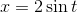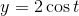from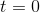to.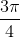Explanation:

There are several ways to solve this problem, but the most effective would be to notice that we can derive the following-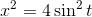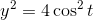Hence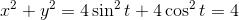Therefore our curve is a circle of radius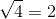, and it's circumfrence is. But we are only interested in half that circumfrence (is fromto, not.), so our answer is.

Alternatively, we could've found the length using the formula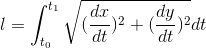.

### Example Question #2 : Parametric Curves

Find the coordinates of the curve function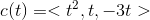when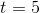.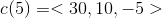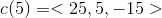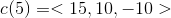Explanation:

To find the coordinates, we setinto the curve function.

We get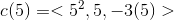and thus### Example Question #3 : Parametric Curves

Find the coordinates of the curve function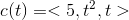when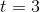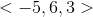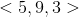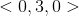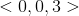Explanation:

To find the coordinates, we evaluate the curve function forAs such,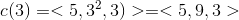### Example Question #4 : Parametric Curves

Find the coordinates of the curve function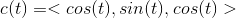when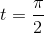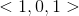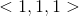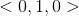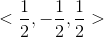Explanation:

To find the coordinates, we evaluate the curve function for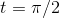As such,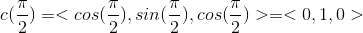### Example Question #5 : Parametric Curves

Find the equation of the line passing through the two points, given in parametric form: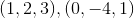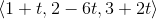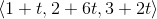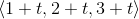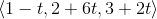Explanation:

To find the equation of the line passing through these two points, we must first find the vector between them: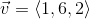This was done by finding the difference between the x, y, and z components for the vectors. (This can be done in either order, it doesn't matter.)

Now, pick a point to be used in the equation of the line, as the initial point. We write the equation of line as follows: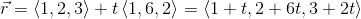The choice of initial point is arbitrary.

### Example Question #6 : Parametric Curves

Find the coordinate of the parametric curve when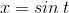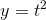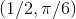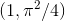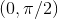Explanation:

To find the coordinates of the parametric curve we plug in for.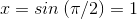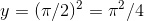As such the coordinates are